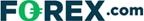Disclosure: Your support helps keep Commodity.com running! We earn a referral fee for some brokers & services we list on this page. Learn more...

# Intro To Linear Regressions Lines – Find Out How They Complement Other Indicators

Last Updated:

In this illustrated guide to understanding the Linear Regression Line, we’ll show you what this chart looks like, explain how to interpret it, and discuss caveats when using it.

## What Is a Linear Regression Line?

A Linear Regression Line is a straight line that best fits the prices between a starting price point and an ending price point.

A “best fit” means that a line is constructed where there is the least amount of deviation between the price points and the actual Linear Regression Line.

## What Is the Linear Regression Line Used For?

The Linear Regression Line is mainly used to determine trend direction. A chart of AT&T (T) stock is given below:

## Interpreting the Linear Regression Line Chart

Traders usually view the Linear Regression Line as the fair value price for futures, stocks, or forex currency pairs.

When prices deviate above or below, traders may expect prices to move back towards the Linear Regression Line.

As a consequence, when prices are below the Linear Regression Line, this could be viewed as a good time to buy, and when prices are above the Linear Regression Line, a good time sell.

## Caveats

Of course, the Linear Regression Line is a very simple test. Other technical indicators would be used to confirm these crude buy and sell signals.

A useful technical analysis charting indicator that uses a Linear Regression Line is the Linear Regression Channel, which gives more objective potential buy and sell signals based on price volatility.

## Where Can I Trade?

Start your research with reviews of these regulated brokers available in . Many have free demo accounts that let you practice your technical trading skills.

CFDs are complex instruments and come with a high risk of losing money rapidly due to leverage. Between 74%-89% of retail investor accounts lose money when trading CFDs. You should consider whether you can afford to take the high risk of losing your money.

Also see our full guides to finding crypto broker or forex broker.

## FAQ

Below we answer some common questions about Linear Regression Line indicators.

### What does regression mean?

In statistics and technical charting, regression is a mathematical description of the relationship between an output variable (like stock price) based on any number of input variables (like dates). The word “regression” implies that the equation is “looking back” at previous prices as a means of predicting future price movements.

Learn more about technical analysis indicators, concepts, and strategies including:

### Plus500 is not available in the US

Legitimate CFD brokers, like Plus500, cannot accept US clients by law## US traders welcome at these brokers:

### Cryoptocurrencies:• Trade 14+ major crypto coins
• Includes Bitcoin, Ethereum & Ripple
• Super simple setup

Accepts traders in the USA

### Forex, Gold & Silver:• Trade gold and silver
• Trade over 90+ currencies
• Major US broker

Accepts traders in the USA

No thanks